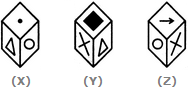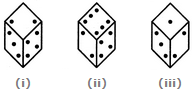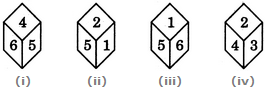# Non Verbal Reasoning - Cubes and Dice

### Exercise :: Cubes and Dice - Section 2

36.

A cube has six different symbols drawn over its six faces. The symbols are dot, circle, triangle, square, cross and arrow. Three different positions of the cube are shown in figures X, Y, and Z.

Which symbol is opposite the arrow?A. Circle B. Triangle C. Dot D. Cross

Answer: Option B

Explanation:

From figures X and Y, we conclude that dot, circle, square and cross lie adjacent to the triangle. Therefore, the arrow must lie opposite the triangle. From figures X and Z, we conclude that dot, triangle, arrow and cross lie adjacent to the circle. Therefore, the square must lie opposite the circle. Thus, the arrow lies opposite the triangle, the square lies opposite the circle and consequently, the cross lies opposite the dot.

As analysed above, the symbol opposite the arrow is the triangle.

37.

Given below are three different positions of a dice. Find the number of dots on the face opposite the face bearing 3 dots.A. 4 B. 5 C. 6 D. Cannot be determined

Answer: Option C

Explanation:

From figures (i), (ii) and (iii), we conclude that 3, 4, 6 and 1 dots appear adjacent to 2 dots. Therefore, 5 dots appear opposite 2 dots. Now, from figures (i) and (iii), we conclude that 2, 4 and 1 dots appear adjacent to 3 dots. Therefore, either 5 or 6 dots appear opposite 3 dots. Since, 5 dots appear opposite 2 dots, it follows that 6 dots appear opposite 3 dots.

38.

Which number is on the face opposite 4, if the four different positions of a dice are as shown in the figure given below.A. 5 B. 3 C. 2 D. 1

Answer: Option D

Explanation:

From figures (i) and (iv) we conclude that 6, 5, 2 and 3 lie adjacent to 4. It follows that 1 lies opposite 4.

39.

A cube has six different symbols drawn over its six faces. The symbols are dot, circle, triangle, square, cross and arrow. Three different positions of the cube are shown in figures X, Y, and Z.

Which symbol occurs at the bottom of fig.(Y)?A. Arrow B. Triangle C. Circle D. Dot

Answer: Option C

Explanation:

From figures X and Y, we conclude that dot, circle, square and cross lie adjacent to the triangle. Therefore, the arrow must lie opposite the triangle. From figures X and Z, we conclude that dot, triangle, arrow and cross lie adjacent to the circle. Therefore, the square must lie opposite the circle. Thus, the arrow lies opposite the triangle, the square lies opposite the circle and consequently, the cross lies opposite the dot.

Since the square lies at the top of fig. (Y) and the circle lies opposite the square (as analysed above), so the circle occurs at the bottom of fig. (Y).

40.

A dice is numbered from 1 to 6 in different ways.

If 1 is adjacent to 2, 4 and 6, then which of the following statements is necessarily true?

 A. 2 is opposite to 6 B. 1 is adjacent to 3 C. 3 is adjacent to 5 D. 3 is opposite to 5

Answer: Option C

Explanation:

If 1 is adjacent to 2, 4 and 6 then either 3 or 5 lies opposite to 1. So, the numbers 3 and 5 cannot lie opposite to each other. Hence, 3 is adjacent to 5 (necessarily).

#### Current Affairs 2021

Interview Questions and Answers